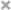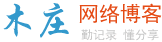# JavaScript判断一个对象是否为数组的几种方法（总结）JS 中的非原始数据类型都是对象（函数具有自己的类型，但它们也是对象）。 因此，仅使用`typeof`运算符来判断是不够的：

 12345 `let result = { subject: ``'Science'``, marks: 97 };``let numbers = [1, 2, 3, 4, 5];` `console.log(``typeof` `result); ``// Object``console.log(``typeof` `numbers); ``// Object`

## 使用 Array.isArray() 方法

 123456789 `let result = { subject: ``"Science"``, marks: 97 }; ``// Object``let numbers = [1, 2, 3, 4, 5]; ``// Array``let name = ``"Mark"``; ``// String``let names = ``new` `Array(``"Jill"``, ``"Jane"``, ``"Jacqueline"``);` `console.log(Array.isArray(result)); ``// false``console.log(Array.isArray(numbers)); ``// true``console.log(Array.isArray(name)); ``// false``console.log(Array.isArray(names)); ``// true`

## 使用对象的构造函数属性

 123456789 `let result = { subject: ``"Science"``, marks: 97 };``let numbers = [1, 2, 3, 4, 5];``let name = ``"Mark"``;``let names = ``new` `Array(``"小智"``, ``"小力"``, ``"小吴"``);` `console.log(result.constructor === Array); ``// false``console.log(numbers.constructor === Array); ``// true``console.log(name.constructor === Array); ``// false``console.log(names.constructor === Array); ``// true`

## 使用 instanceof 运算符

`instanceof`运算符检查是否在对象的原型链中找到构造函数。

`typeof`运算符一样，它返回布尔值。 要确定变量是否为数组，可以使用`instanceof`，如下所示：

 123456789 `let result = { subject: ``"Science"``, marks: 97 };``let numbers = [1, 2, 3, 4, 5];``let name = ``"Mark"``;``let names = ``new` `Array(``"小智"``, ``"小力"``, ``"小吴"``);` `console.log(result ``instanceof` `Array); ``// false``console.log(numbers ``instanceof` `Array); ``// true``console.log(name ``instanceof` `Array); ``// false``console.log(names ``instanceof` `Array); ``// true`

## 使用 Object.prototype.call() 方法

JS 中的所有对象均从主原型对象继承属性，该对象命名为`Object.prototype``Object.prototype`中存在`toString()`方法，这是每个对象都有自己的`toString()`方法的原因， `Object.prototype``toString()`方法显示对象的类型。

 1234567891011121314 `let result = { subject: ``"Science"``, marks: 97 };``let numbers = [1, 2, 3, 4, 5];``let name = ``"Mark"``;``let names = ``new` `Array(``"小智"``, ``"小力"``, ``"小吴"``);` `console.log(Object.prototype.toString.call(result)); ``// [object Object]``console.log(Object.prototype.toString.call(numbers)); ``// [object Array]``console.log(Object.prototype.toString.call(name)); ``// [object String]``console.log(Object.prototype.toString.call(names)); ``// [object Array]` `console.log(Object.prototype.toString.call(result) === ``"[object Array]"``); ``// false``console.log(Object.prototype.toString.call(numbers) === ``"[object Array]"``); ``// true``console.log(Object.prototype.toString.call(name) === ``"[object Array]"``); ``// false``console.log(Object.prototype.toString.call(names) === ``"[object Array]"``); ``// true`

## 总结

java和javascript有什么区别

javascript数组怎么删除项（元素）

javascript是什么技术

javascript今年25 岁了！

javascript中回文数是什么？怎么判断？

javascript由哪个公司创建

javascript如何删除div标签

10 种快速优化 web 性能的手段（分享）

javascript中的运算符有哪些

8个编写优秀js代码的技巧和窍门（分享）### 评论

• 欢迎访问木庄网络博客
• 可复制：代码框内的文字。
• 方法：Ctrl+C。